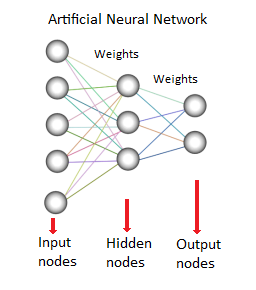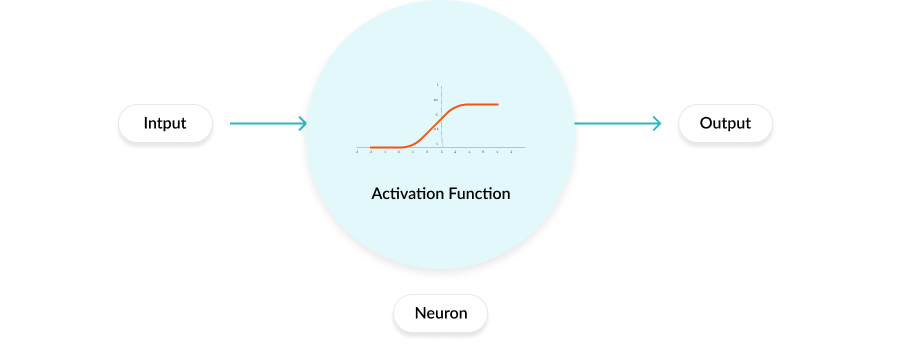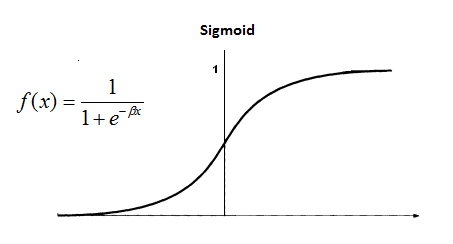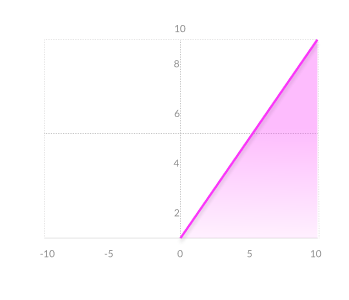HomeBlogArtificial IntelligenceHow to Make a Neural Network: Architecture, Parameters & Code

# How to Make a Neural Network: Architecture, Parameters & Code

Last updated:
12th Feb, 2021
Views
8 MinsView AllNeural Networks as the name suggests are circuits of Neurons. There are different types of Neural Networks. Biological Neural Networks are made of real biological Neurons. Whereas Artificial Neural Networks (ANN) is a system that is based on the biological Neural Network, like present in the brain. The estimated number of Neurons in the brain are around 100 BIllion, which communicate through electrochemical signals.

## Top Machine Learning and AI Courses Online

 Master of Science in Machine Learning & AI from LJMU Executive Post Graduate Programme in Machine Learning & AI from IIITB Advanced Certificate Programme in Machine Learning & NLP from IIITB Advanced Certificate Programme in Machine Learning & Deep Learning from IIITB Executive Post Graduate Program in Data Science & Machine Learning from University of Maryland To Explore all our certification courses on AI & ML, kindly visit our page below. Machine Learning Certification

The ANN tries to recreate the computational complexity present in the biological Neurons but it’s not as comparable to that and they are much simpler and non-complex versions of biological neural networks. In this article, we’ll understand the structure of an ANN and learn how to create a Neural Network using Python.

## Trending Machine Learning Skills

 AI Courses Tableau Certification Natural Language Processing Deep Learning AI

Learn ML Course from the World’s top Universities. Earn Masters, Executive PGP, or Advanced Certificate Programs to fast-track your career.

## Neural Network Architecture

The artificial neural network is made up of artificial neurons which are also called “Nodes”. These nodes are connected to each other such that a network or mesh is created. The strength of these connections to one another is assigned a value. This value lies between -1 to 1.

If the value of the connection is high it indicates a strong connection between those nodes. Every node has a characteristic function to it. Changing this function will change the behaviour and complexity nature of the neural network. There are three types of neurons in an ANN, input nodes, hidden nodes, and output nodes as shown below:Source

The input node is responsible for receiving the information which is generally in the form of numerical values or expressions. The information is presented as activation values, where each node is given a number, the higher the number, the greater the activation.
The information is further passed down the network. Based on the Node connection weights and the activation function pertaining to the certain neurons of specific layers, the information is passed on from neuron to neuron. Each of the nodes adds the activation values upon receival, the values are modified on the basis of the transfer function.
The information flows throughout the network, through hidden layers, until it reaches the output nodes. The output nodes are very important as they reflect the input in a meaningful way to the outside world. Here an amazing aspect of neural networks can be seen which leads to the adjustment of weights for every layer and nodes.

The difference between the predicted value and the actual value (error) will be propagated backwards. The Neural Network hence will learn from the errors made and try to adjust the weights on the basis of the designated learning rate approach.

Hence by adjusting the parameters like a number of hidden layers, a number of neurons per layer, weight updation strategy and activation function, we can create a Neural Network.

## Define The Parameters

### Activation Function

There are various activation functions to choose from that can be used in the Neural Network on the basis of the problem in hand.

Activation functions are mathematical equations that every neuron has. It determines the output of a Neural Network.
This activation function is attached to every neuron in the network and determines if it should be activated or not, which is based on if the activation of that particular neuron helps in deriving relevant predictions at the output layer. Different layers can have different activation functions attached to it. Activation functions also help normalize the output of each neuron to a range between 1 and 0 or between -1 and 1.

Modern neural networks use an important technique called backpropagation to train the model by adjusting the weights, which places an increased computational strain on the activation function, and its derivative function.Working of an activation function

There are 3 types of Activation functions:
Binary- x<0 y=0 , x>0 y=1
Linear- x=y
Non Linear – Various types : Sigmoid, TanH, Logistic, ReLU,Softmax etc.Source: BlogType: ReLU

### Algorithm

There are many types of neural networks, but they are often divided into feed-forward and feed-back (backpropagation) networks.

1) The forward feed network is a non-repetitive network that contains inputs, outputs, and hidden layers; as the signals can only move in one direction. The input data is transferred to the processing equipment layer where it performs the calculations. Each processing factor makes its calculation based on the weight of the input. New values ​​are calculated and then new input values ​​feed the next layer.

This process continues until it passes through all the layers and determines the outcome. A Limit transfer function is sometimes used to measure neuron output in the output layer. Feed Forward networks are known as and include Perceptron (direct and indirect) networks. Feed-forward networks are often used for data mining.

2) The Feed-Back network (e.g., a recurrent neural network or RNN) has retrospective mechanisms which means they can have signals moving in both directions using traps/loops. All possible communication between neurons is allowed.

Since the loops are present in this type of network, it becomes a nonlinear system that is constantly changing until it reaches a state of stability. Feed-back networks are often used for memories associated with performance problems when the network is looking for a good set of connected objects.

### Training

the feed-forward pass means given an input and weights how the output is computed. After training completion, we only run the forward pass to form the predictions.

But we first got to train our model to truly learn the weights, and therefore the training procedure works as follows:

1. Randomly select and initialise the weights for all the nodes. There are smart initialization methods which are inbuilt in TensorFlow and Keras (Python).
2. For every training example, perform a forward pass using the present weights, and calculate the output of every node going from left to right. The ultimate output is the value of the last node.
3. Compare the final output with the actual target within the training data, and measure the error employing a loss function.
4. Perform a backwards pass from right to left and propagate the error calculated in the last step to each individual node using backpropagation.
5. Calculate each neuron’s weight contribution to the error, and adjust the weights of the connection accordingly using gradient descent. Propagate the error gradients back ranging from the last layer.

## Python Code for Neural Network

Now that we understand how Neural Network is made Theoretically, let us implement the same using Python.

Neural Network in Python
We will use the Keras API with Tensorflow or Theano backends for creating our neural network.

Installing libraries
Theano
>>> pip install –upgrade –no-deps git+git://github.com/Theano/Theano.git

Tensorflow and Keras
>>> pip3 install tensorflow

Import the libraries

import keras
from keras.models import Sequential
from keras.layers import Dense

Initialising the Artificial Neural Network

model = Sequential()

Creates Input and Hidden Layers-

model.add(Dense(input_dim = 2, units = 10, activation=’relu’, kernel_initializer=’uniform’))

This code adds the input layer and one hidden layer to the sequential network
Dense(): lets us create a densely connected neural network
input_dim: shape or number of nodes in the input layer
units: the number of neurons or nodes in the current layer (hidden layer)
activation: the activation function applied to each node.”relu” stands for Rectified Linear Unit
kernel_initializer: initial random weights of the layer

Second hidden layer

The code creates and adds another hidden layer to the model with 20 nodes and ‘rectified Linear’ activation function. More layers can be added in a similar way depending on the problem and the complexity.

Output Layer

A single output layer with Sigmoid or softmax are the commonly used activation functions for an output layer.

ANN compilation:

The ANN is compiled with an optimizer function and a loss function before being trained.

Optimizer: an optimizer function for the network, There are various types of optimizers and adam is mostly used.
Loss: used for calculating the losses and errors. There are various types and the choice depends on the nature of the problem being dealt.
Metrics: the metric used to measure the performance of the model.

Fitting the model with the training data:
model.fit(X_train,Y_train,batch_size=64, epochs=30)

This code will create the model

## Popular AI and ML Blogs & Free Courses

 IoT: History, Present & Future Machine Learning Tutorial: Learn ML What is Algorithm? Simple & Easy Robotics Engineer Salary in India : All Roles A Day in the Life of a Machine Learning Engineer: What do they do? What is IoT (Internet of Things) Permutation vs Combination: Difference between Permutation and Combination Top 7 Trends in Artificial Intelligence & Machine Learning Machine Learning with R: Everything You Need to Know AI & ML Free Courses Introduction to NLP Fundamentals of Deep Learning of Neural Networks Linear Regression: Step by Step Guide Artificial Intelligence in the Real World Introduction to Tableau Case Study using Python, SQL and Tableau

## Conclusion

We can now create an Artificial Neural Network (on Python) from scratch as we understood the different parameters that can be changed according to the problem in hand.

If you’re interested to learn more about deep learning techniquesmachine learning, check out IIIT-B & upGrad’s PG Diploma in Machine Learning & AI which is designed for working professionals and offers 450+ hours of rigorous training, 30+ case studies & assignments, IIIT-B Alumni status, 5+ practical hands-on capstone projects & job assistance with top firms.Blog Author
Director of Engineering @ upGrad. Motivated to leverage technology to solve problems. Seasoned leader for startups and fast moving orgs. Working on solving problems of scale and long term technology strategy.
Get Free Consultation

Select CourseSelectBy clicking 'Submit' you Agree to

## Suggested Blogs

91070
AWS Projects & Topics Looking for AWS project ideas? Then you’ve come to the right place because, in this article, we’ve shared multiple AWS proj